# Machine Learning - Lecture 4: The Naive Bayes Classifier

Chris Thornton

```  SYMPTOM       OCCUPATION    AILMENT

sneezing      nurse         flu
sneezing      farmer        hayfever
sneezing      teacher       flu
```
```  sneezing      builder       ???
```

What ailment should we predict for a sneezing builder and why?

# Introduction

Clustering and nearest-neighbour methods are ideally suited for use with numeric data.

However, data often use using categorical values, i.e., names or symbols.

In this situation, it may be better to use a probabilistic method, such as the Naive Bayes Classifier (NBC).

# Probabilities

Let's say we have some data that lists symptoms and ailments for everybody in a certain group.

```  SYMPTOM       AILMENT

sneezing      flu
sneezing      hayfever
sneezing      flu
coughing      flu
backache      none
vomiting      concussion
crying        hayfever
temperature   flu
drowsiness    concussion
```

# Prediction

There are 10 cases in all so we can work out the probability of seeing a particular ailment or symptom just by counting and dividing by 10.

```  P(hayfever) = 2/10 = 0.2

P(vomiting) = 1/10 = 0.1
```
As a simple, statistical model of the data, these (so-called prior) probabilities can be used for prediction.

Let's say we're undecided whether someone has flu or hayfever.

We can use the fact that P(flu) > P(hayfever) to predict it's more likely to be flu.

# Conditional probabilities

This sort of modeling becomes more useful when conditional probabilities are used.

These are values we work out by looking at the probability of seeing one value given we see another, e.g., the probability of vomiting given concussion.

Conditional probabilites are notated using the bar `|' to separate the conditioned from the conditioning value.

The probability of vomiting given concussion is written

```  P(vomiting|concussion)
```
We can work this value out by seeing what proportion of the cases involving concussion also show vomiting.

```  P(vomiting|concussion) = 1/3 = 0.3333
```

# Prediction from conditional probabilities

Conditional probabilities enable conditional predictions.

For example, we could tell someone who's known to have concussion that there's a 1/3 chance of them vomiting.

This can also be a way of generating diagnoses.

If someone reports they've been sneezing a lot, we can say there's a 2/3 chance of them having flu, since

```  P(flu|sneezing) = 2/3
```
With slightly less likelihood (1/3) we could say they have hayfever, since

```  P(hayfever|sneezing) = 1/3
```

# The problem of multiple attributes

What happens if the data include more than one symptom?

We might have something like this.

```  SYMPTOM       OCCUPATION    AILMENT

sneezing      nurse         flu
sneezing      farmer        hayfever
```
We'd like to be able to work out probabilities conditional on multiple symptoms, e.g.,

```  P(flu|sneezing,builder)
```
But if a combination doesn't appear in the data, how do we calculate its conditional probability?

# Using inversion

There's no way to sample a probability conditional on a combination that doesn't appear.

But we can work it out by looking at probabilities that do appear.

Observable probabilities that contribute to

```  P(flu|sneezing,builder)
```
are

```  P(flu)
P(sneezing|flu)
P(builder|flu)
```
All we need is some way of putting these together.

# The naive assumption

Probability theory says that if several factors don't depend on each other in any way, the probability of seeing them together is just the product of their probabilities.

So assuming that sneezing has no impact on whether you're a builder, we can say that

```  P(sneezing,builder|flu) = P(sneezing|flu)P(builder|flu)
```
The probability of a sneezing builder having flu must depend on the chances of this combination of attributes indicating flu. So

```  P(flu|sneezing,builder)
```
must be proportional to

```  P(flu)P(sneezing,builder|flu)
```

# Normalization needed

Unfortunately, this value is purely based on cases of flu. It doesn't take into account how common this ailment is.

We need to factor in the probability of this combination of attributes associating with flu in particular, rather than some other ailment.

We do this by expressing the value in proportion to the probability of seeing the combination of attributes.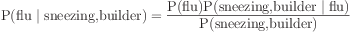This gives us the value we want.

Assemble all the constituents needed

P(flu) = 0.5
P(sneezing|flu)=0.66
P(builder|flu)=0.33
P(sneezing,builder|flu)=(0.66x0.33)=0.22
P(sneezing)=0.5
P(builder)=0.33
P(sneezing,builder)=(0.5x0.33)=0.165
Plug values into the formula: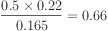It turns out the sneezing builder has flu with probability 0.66.

# Bayes rule

What we've worked out here is just an application of Bayes rule, the standard formula for inverting conditional probabilities.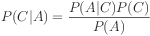We've looked at ailments and symptoms, but the method can be used whenever we need classifications of cases described in terms of attributes.

The more general version of Bayes rule deals with the case where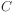is a class value, and the attributes are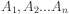.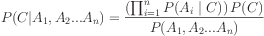# Naive Bayes Classifier

A Naive Bayes Classifier is a program which predicts a class value given a set of set of attributes.

For each known class value,

1. Calculate probabilities for each attribute, conditional on the class value.

2. Use the product rule to obtain a joint conditional probability for the attributes.

3. Use Bayes rule to derive conditional probabilities for the class variable.

Once this has been done for all class values, output the class with the highest probability.

# The problem of missing combinations

A niggling problem with the NBC is where the dataset doesn't provide one or more of the probabilities we need.

We then get a probability of zero factored into the mix.

This may cause us to divide by zero, or simply make the final value itself zero.

The easiest solution is to ignore zero-valued probabilities altogether if we can.

# Idiot's Bayes?

Statisticians are somewhat disturbed by use of the NBC (which they dub Idiot's Bayes) because the naive assumption of independence is almost always invalid in the real world.

However, the method has been shown to perform surprisingly well in a wide variety of contexts.

Research continues on why this is.

# Summary

• Clustering and nearest-neighbour methods ideally suited to numeric data.

• Probablistic modeling may be more effective with categorical (symbolic) data.

• Probabilities easily derived from datasets.

• But for classification, we normally need to invert the conditional probabilities we can sample.

• The Naive Bayes Classifier uses Bayes Rule to identify the class with the highest probability.

• On average, the NBC seems to be perform better than expected.

# Questions

• What domain do the probabilities we derive from a dataset apply to?

• What is the difference between a conditioning and a conditioned value in a defined probability?

• Where should we place the conditioned value in a conditional probability statement?

• What sort of modeling process is involved in the NBC?

• Where we have just one class, and one attribute variable, we can work out all conditional probabilities directly from the dataset. Why is this more difficult with more than one attribute?

• Identify two attributes that are certainly independent, two that are certainly dependent, and two that are somewhere in between.